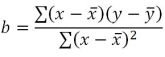# SQL Server SLOPE function

Updated: 9 August 2010

Use SLOPE_q to calculate the slope of the linear regression through the data points in the known x-values and y-values. The slope is the vertical distance divided by the horizontal distance between any two points on the line, which is the rate of change along the regression line. The equation for the slope of the regression line is:Syntax
SELECT [wctStatistics].[wct].[SLOPE_q] (
<@Known_y_Known_x_RangeQuery, nvarchar(4000),>)
Arguments

@Known_y_Known_x_RangeQuery
the select statement, as text, used to determine the known y- and x-values to be used in the SLOPE_q function.
Return Types
float
Remarks
·         If the number of y-data points is not equal to the number of x-data points, SLOPE_q will return an error.
·         No GROUP BY is required for this function even though it produces aggregated results.
·         For simpler queries, use the SLOPE function.
Examples
CREATE TABLE #i1(
[y] [float] NOT NULL,
[x] [float] NOT NULL
)
INSERT INTO #i1 VALUES (-4.5,-1)
INSERT INTO #i1 VALUES (0,2)
INSERT INTO #i1 VALUES (-9,-4)
INSERT INTO #i1 VALUES (4.5,5)
INSERT INTO #i1 VALUES (-13.5,-7)

SELECT
wct.SLOPE_q('SELECT y, x from #i1')

This produces the following result
----------------------
1.5

(1 row(s) affected)

### SupportCopyright 2008-2023 Westclintech LLC         Privacy Policy        Terms of Service